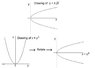# Why are these graphs so different? y^2=x y=sqrt(x)

• noahsdev
In summary, the conversation discusses the difference between the graphs of y2=x and y=√x. The two graphs are not the same and require different plotting methods due to the function of the drawing tool. The conversation also mentions the importance of not forgetting the sign in front of the square root. Lastly, there is a discussion about the appropriate way to plot the graph, with a mention of rotating the sheet of paper to properly align the axes. The conversation ends with a resolution and understanding of the topic.

#### noahsdev

Why are the graphs of y2=x and y=√x so different? They're supposed to be the same, aren't they? I'm usually good at maths but I honestly don't understand this. Can someone explain please? Thanks :).

They are not different.
But do not forget the sign (+or-) in front of the square root.
And you have to plot x=y², not y=x². Since your drawing tool draws the ordinate as a function of the abscissa and that you have to plot x as a function of y (not y as a function of x as usual), you have to rotate the sheet of paper (the axes) of 90°

#### Attachments

•Plot.JPG
18.1 KB · Views: 586
Last edited:
JJacquelin said:
They are not different.
But do not forget the sign (+or-) in front of the square root.
And you have to plot x=y², not y=x². Since your drawing tool draws the ordinate as a function of the abscissa and that you have to plot x as a function of y (not y as a function of x as usual), you have to rotate the sheet of paper (the axes) of 90°

Thankyou! They are different, but your comment helped me understand why :). The y=√x is only the positive side, but when you simplify the x=y2, expression you have to add the ± to the square root. Thanks again.

#### Attachments

JJacquelin said:
They are not different.
But do not forget the sign (+or-) in front of the square root.
And you have to plot x=y², not y=x². Since your drawing tool draws the ordinate as a function of the abscissa and that you have to plot x as a function of y (not y as a function of x as usual), you have to rotate the sheet of paper (the axes) of 90°
Call me a pedant, but generally you shouldn't "rotate the graph" - you should "reflect the graph" in the line y=x. In this particular case it happens to produce the same result.noahsdev said:
Why are the graphs of y2=x and y=√x so different? They're supposed to be the same, aren't they? I'm usually good at maths but I honestly don't understand this. Can someone explain please? Thanks :).

JJacquelin said:
They are not different.
The two graphs ARE different. The graph of y =√x is the upper half of the graph of y2 = x
noahsdev said:
But do not forget the sign (+or-) in front of the square root.
And you have to plot x=y², not y=x². Since your drawing tool draws the ordinate as a function of the abscissa and that you have to plot x as a function of y (not y as a function of x as usual), you have to rotate the sheet of paper (the axes) of 90°

Hi !
The figure in my preceeding post was there in order to explain what I mean, better than with words.
Noahsdev said that he understood it.
All's well that ends well.